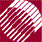ECE532 Biomedical Optics © 1998 Steven L. Jacques, Scott A. Prahl Oregon Graduate Institute

# Diffusion theory

## Introduction

Diffusion theory is the modeling of photon transport due to photon movement down concentration gradients. Diffusion theory is appropriate in medium dominated by scattering rather than absorption so that each photon undergoes many scattering events before being terminated by an absorption event. The photon has a relatively long residence time which allows the photon to engage in a random walk within the medium.

Diffusion theory = Photonsfallingdownagradientof photon concentration.

The instantaneous fluence rate, F(r,t) [J s-1 cm-2] or [W cm-2], is proportional to the concentration of optical energy, C(r,t) [J cm-3] and the speed of light, c [cm/s], in the medium:

## F(r,t) = cC(r,t)

The units of energy concentration [J cm-3] times the units of velocity [cm/s] yield the units of fluence rate [J s-1 cm-2]. This relationship between F and C allows us to discuss the time-resolved spatial distribution of photon concentration, C(r,t), in a light-scattering medium using the same math of diffusion which applies to many things such as solutes in a solution or heat in a material.

to next page | Chapter 5 | Course | Home